### Linear Equation In Two Variables Class 9th Mathematics AP Board Solution

##### Class 9th Mathematics AP Board Solution

###### Exercise 6.3
Question 1.

Draw the graph of each of the following linear equations.

2y = -x + 1

For graph, we’ll first make the table of solutions by putting some random values of x and thereafter we’ll find corresponding values of y and then we’ll plot these points on graph, join them and extend them in straight line to find the graph.

(Note: ∵ equation is linear graph will always be straight line.)

Table of solutions for the given equation-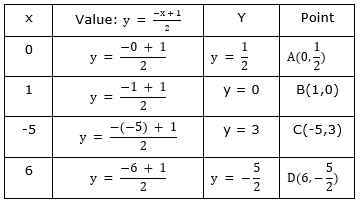GRAPH: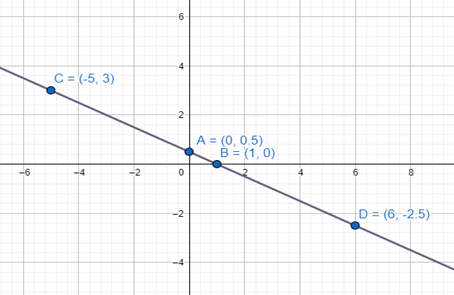Question 2.

Draw the graph of each of the following linear equations.

–x + y = 6

For graph, we’ll first make the table of solutions by putting some random values of x and thereafter we’ll find corresponding values of y and then we’ll plot these points on graph, join them and extend them in straight line to find the graph.

(Note: ∵ equation is linear graph will always be straight line.)

Table of solutions for the given equation-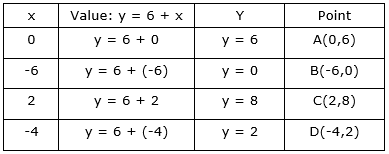GRAPH: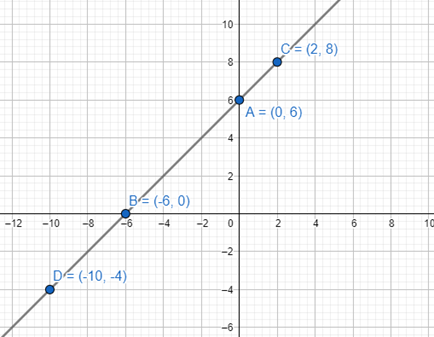Question 3.

Draw the graph of each of the following linear equations.

3x + 5y = 15

For graph, we’ll first make the table of solutions by putting some random values of x and thereafter we’ll find corresponding values of y and then we’ll plot these points on graph, join them and extend them in straight line to find the graph.

(Note: ∵ equation is linear graph will always be straight line.)

Table of solutions for the given equation-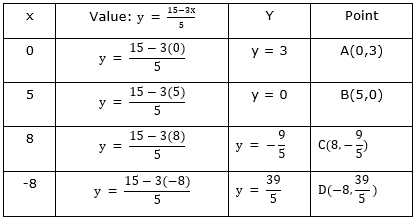GRAPH: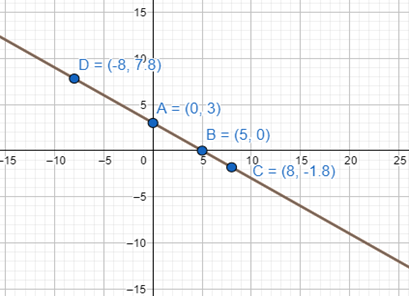For graph, we’ll first make the table of solutions by putting some random values of x and thereafter we’ll find corresponding values of y and then we’ll plot these points on graph, join them and extend them in straight line to find the graph.

(Note: ∵ equation is linear graph will always be straight line.)

Table of solutions for the given equation-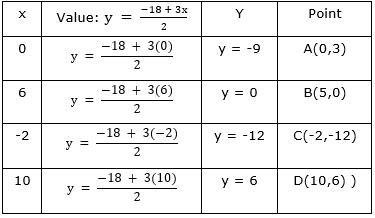GRAPH: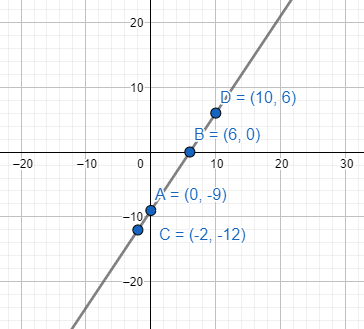Question 5.

Draw the graph of each of the following linear equations.

y = x

For graph, we’ll first make the table of solutions by putting some random values of x and thereafter we’ll find corresponding values of y and then we’ll plot these points on graph, join them and extend them in straight line to find the graph.

(Note: ∵ equation is linear graph will always be straight line.)

Table of solutions for the given equation-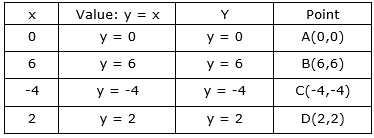GRAPH: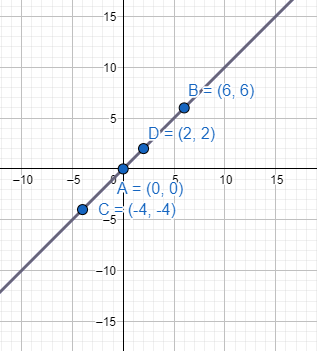Question 6.

Draw the graph of each of the following linear equations.

y = 2x

For graph, we’ll first make the table of solutions by putting some random values of x and thereafter we’ll find corresponding values of y and then we’ll plot these points on graph, join them and extend them in straight line to find the graph.

(Note: ∵ equation is linear graph will always be straight line.)

Table of solutions for the given equation-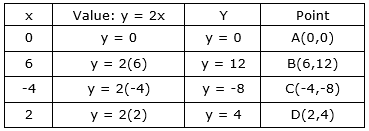GRAPH: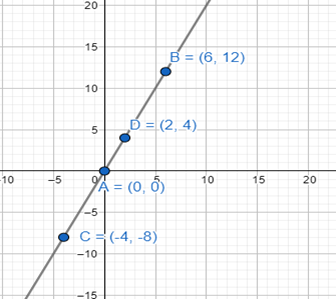Question 7.

Draw the graph of each of the following linear equations.

y = -2x

For graph, we’ll first make the table of solutions by putting some random values of x and thereafter we’ll find corresponding values of y and then we’ll plot these points on graph, join them and extend them in straight line to find the graph.

(Note: ∵ equation is linear graph will always be straight line.)

Table of solutions for the given equation-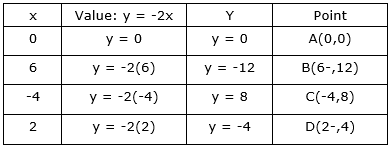GRAPH: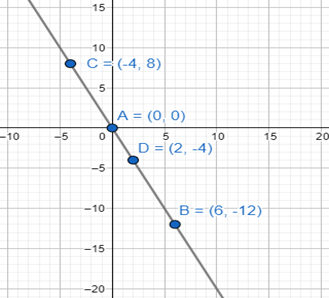Question 8.

Draw the graph of each of the following linear equations.

y = 3x

For graph, we’ll first make the table of solutions by putting some random values of x and thereafter we’ll find corresponding values of y and then we’ll plot these points on graph, join them and extend them in straight line to find the graph.

(Note: ∵ equation is linear graph will always be straight line.)

Table of solutions for the given equation-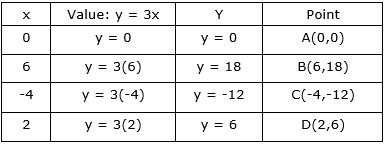GRAPH: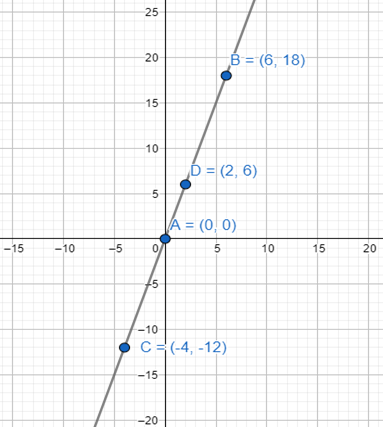Question 9.

Draw the graph of each of the following linear equations.

y = -3x

For graph, we’ll first make the table of solutions by putting some random values of x and thereafter we’ll find corresponding values of y and then we’ll plot these points on graph, join them and extend them in straight line to find the graph.

(Note: ∵ equation is linear graph will always be straight line.) Table of solutions for the given equation-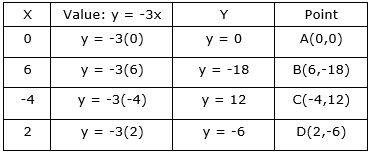GRAPH: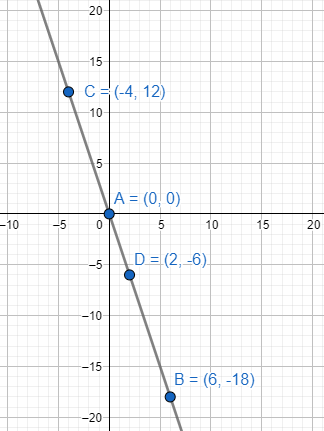Question 10.

Answer the following question related to above graphs.

i) Are all these equations of the form y = mx, where m is a real number?

ii) Are all these graphs passing through the origin?

iii) What can you conclude about these graphs?

(i) Yes, all these are equations of the form y = mx, where m is a real number and m = 1,2,-2,3,-3 respectively in the above equations.

(ii) Yes, all these are graphs passing through the origin, i.e., pt. A in every graph

(iii) ∴ we can conclude that every graph of type y = mx passes through origin, where m is a real number.

Question 11.

Draw the graph of the equation 2x + 3y = 11. Find from the graph value of y when x = 1

For graph, we’ll first make the table of solutions by putting some random values of x and thereafter we’ll find corresponding values of y and then we’ll plot these points on graph, join them and extend them in straight line to find the graph.

(Note: ∵ equation is linear graph will always be straight line.)

Table of solutions for the given equation-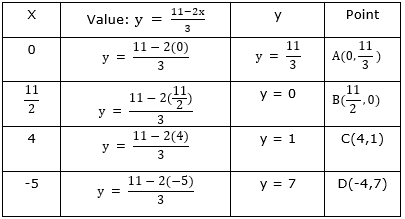GRAPH: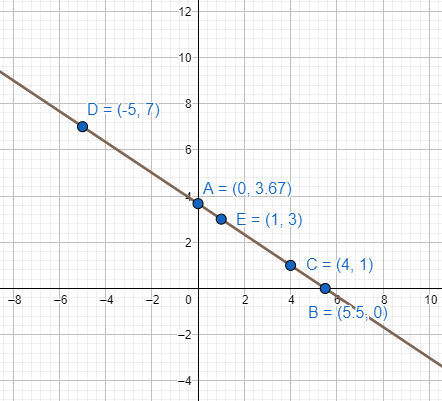From the graph (pt. E) we can see that for x = 1, the y = 3.

(Note: Also we can put x = 1 in the given equation and can find the value of y-

We have,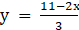At x = 1,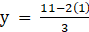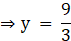⇒ y = 3

Question 12.

Draw the graph of the equation y - x = 2. Find from the graph

i) the value of y when x = 4

ii) the value of x when y = -3

For graph, we’ll first make the table of solutions by putting some random values of x and thereafter we’ll find corresponding values of y and then we’ll plot these points on graph, join them and extend them in straight line to find the graph.

(Note: ∵ equation is linear graph will always be straight line.)

Table of solutions for the given equation-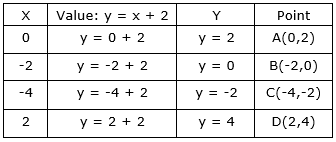GRAPH: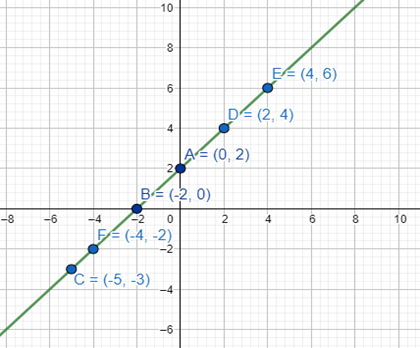i)the value of y when x = 4 is y = 6 (pt. E)

ii) the value of x when y = -3 is y = -5 (pt. F)

Question 13.

Draw the graph of the equation 2x + 3y = 12. Find the solutions from the graph

i) Whose y-coordinate is 3

ii) Whose x-coordinate is -3

For graph, we’ll first make the table of solutions by putting some random values of x and thereafter we’ll find corresponding values of y and then we’ll plot these points on graph, join them and extend them in straight line to find the graph.

(Note: ∵ equation is linear graph will always be straight line.)

Table of solutions for the given equation-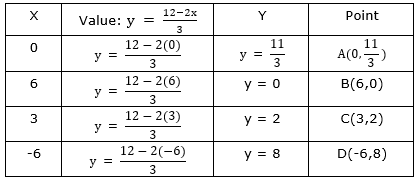GRAPH: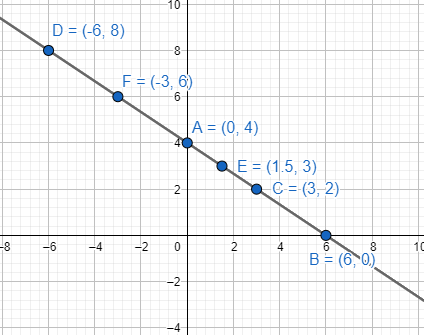(i) From the graph, we can see that for y = 3 is pt. E and the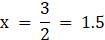(ii) From the graph, we can see that for x = -3 is pt. F and the corresponding y = 6 for that.

Question 14.

Draw the graph of each of the equations given below and also find the coordinates of the points where the graph cuts the coordinate axes

6x - 3y = 12

For graph, we’ll first make the table of solutions by putting some random values of x and thereafter we’ll find corresponding values of y and then we’ll plot these points on graph, join them and extend them in straight line to find the graph.

(Note: ∵ equation is linear graph will always be straight line.)

Table of solutions for the given equation-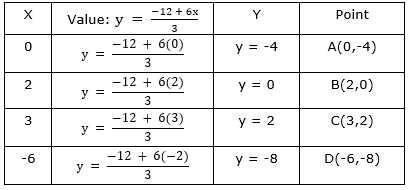GRAPH: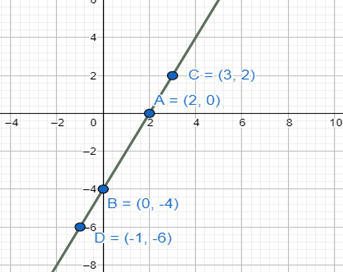⇒ ∴ the pts. Where graph cuts the co-ordinate axis(i.e., where x = 0 and where y = 0) are pt. A = (2,0) and pt. B = (0,-4)

Question 15.

Draw the graph of each of the equations given below and also find the coordinates of the points where the graph cuts the coordinate axes

- x + 4y = 8

For graph, we’ll first make the table of solutions by putting some random values of x and thereafter we’ll find corresponding values of y and then we’ll plot these points on graph, join them and extend them in straight line to find the graph.

(Note: ∵ equation is linear graph will always be straight line.)

Table of solutions for the given equation-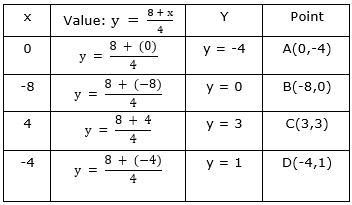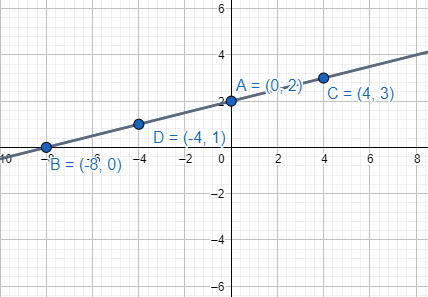⇒ ∴ the pts. Where graph cuts the co-ordinate axis(i.e., where x = 0 and where y = 0) are pt. A = (-8,0) and pt. B = (0,2).

Question 16.

Draw the graph of each of the equations given below and also find the coordinates of the points where the graph cuts the coordinate axes

3x + 2y + 6 = 0

For graph, we’ll first make the table of solutions by putting some random values of x and thereafter we’ll find corresponding values of y and then we’ll plot these points on graph, join them and extend them in straight line to find the graph.

(Note: ∵ equation is linear graph will always be straight line.)

Table of solutions for the given equation-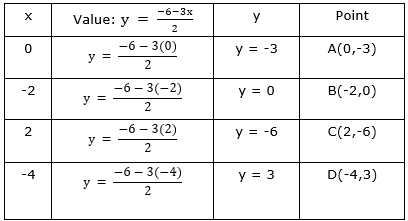GRAPH: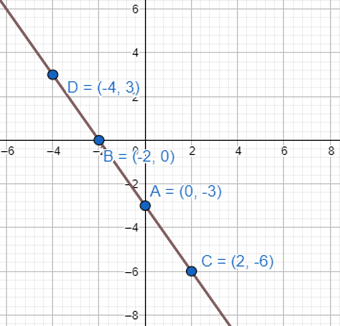⇒ ∴ the pts. Where graph cuts the co-ordinate axis(i.e., where x = 0 and where y = 0) are pt. A = (-2,0) and pt. B = (0,-3).

Question 17.

Rajiya and Preethi two students of Class IX together collected ₹ 1000 for the Prime Minister Relief Fund for victims of natural calamities. Write a linear equation and draw a graph to depict the statement.

Given that together Rajiya and Preethi collected Rs.1000.

Now, Let the amount collected by Rajiya be Rs. x and by Preethi be Rs. y.

∴ the linear equation will be-

⇒ x + y = 1000

For graph, we’ll first make the table of solutions by putting some random values of x and thereafter we’ll find corresponding values of y and then we’ll plot these points on graph, join them and extend them in straight line to find the graph.

(Note: ∵ equation is linear graph will always be straight line.)

Table of solutions for the given equation-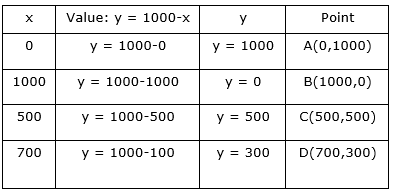GRAPH: